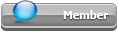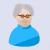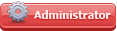## Featured Articles

Check out the latest featured articles.## File Library3# Pressure Increase With Time

17 replies to this topic
|

### #1niki22

niki22

Brand New Member

•• Members
•• 9 posts

Posted 23 November 2022 - 11:43 PM

I am new to this field. how i can find pressure increase with time? for example..if at 0 second pressure is 20 barg with flo 100000kg/hr. then what will be the pressure at 5 sec?

### #2Pilesar

Pilesar

Gold Member

•• Members
•• 1,077 posts

Posted 24 November 2022 - 10:39 AM

Steady state calculations are simpler than dynamic calculations. You might try a series of steady state calculations as an approximation. The info in your post is too sparse to get an idea of what you are really needing.

### #3latexman

latexman

Gold Member

••• 1,470 posts

Posted 24 November 2022 - 11:14 AM

Is this gas flow? If so, and as Pilesar indicated, at 5 seconds how much mass has accumulated in your system volume? Then apply PV = nRT. More details would have been useful, and we wouldn’t have to guess.

### #4niki22

niki22

Brand New Member

•• Members
•• 9 posts

Posted 25 November 2022 - 02:56 PM

yeah it's gas flow. if we use PV=nRT how it will say after eacond how much pressure increase? i need to find out how much seconds we have to close the XV once control valve fails. so need to find step by step time from normal operating to PSV set point

### #5niki22

niki22

Brand New Member

•• Members
•• 9 posts

Posted 25 November 2022 - 03:16 PM

i dont know it is the right way or not but for first step i found mass from kg/hr by multiplying with 1sec. so if 156000 kg/hr flow mass i got 156000/3600 = 43 kg. now how to do it for next step 2sec. i will have to find pressure for this mass first but i dont know how to?

Edited by niki22, 25 November 2022 - 05:16 PM.

### #6Pilesar

Pilesar

Gold Member

•• Members
•• 1,077 posts

Posted 25 November 2022 - 08:28 PM

### #7niki22

niki22

Brand New Member

•• Members
•• 9 posts

Posted 25 November 2022 - 10:31 PM

I am chemical engineer graduate. Basically want to find step by step pressure by consider shutoff off valve(XV) Cv and after how many seconds it will reach to PSV set pressure. i found the total time by below equation..

time=(density@PSV set pressure - density @normal operating pressure)/flow for accumulation.

But for detail report they need step by step pressure increase. i know P=F/A (kg/cm2) and then i can multiply by 0.981 to convert in Bar. but i am not sure is this the right way to do it? i am not confident about it because never done this kind of calculation after i joined as graduate. Can we consider area(A) as pi/4xd2 or need to consider surface area of whole pipe? too many confusions because this is my first ever calc.

Edited by niki22, 25 November 2022 - 10:59 PM.

### #8breizh

breizh

Gold Member

••• 5,696 posts

Posted 25 November 2022 - 11:01 PM

Hi,

Can you sketch your problem for us to better understand?

Do you mean you have a reservoir (volume) at t=0 with a pressure P0 and you want to know the evolution of P of this particular volume over the time?

If you are a student, you should post in the student forum.

Breizh

### #9latexman

latexman

Gold Member

••• 1,470 posts

Posted 25 November 2022 - 11:07 PM

n = PV/RT

P = nRT/V

So you have to know temperature and system volume. Then you know “at 0 second pressure is 20 barg”. Calculate n at 0 second. Then you know 43 kg gets added every second. So you can calculate the pressure at 5 second.

### #10niki22

niki22

Brand New Member

•• Members
•• 9 posts

Posted 25 November 2022 - 11:12 PM

@latexman...how to find that pressure for extra mass added..that is the confusion. from P=F/A? Volume is fixed but without knowing temperature for extra addes mass how i can use P=nRT/V

Edited by niki22, 25 November 2022 - 11:22 PM.

### #11breizh

breizh

Gold Member

••• 5,696 posts

Posted 25 November 2022 - 11:30 PM

Hi,

You know your initial condition (P0, T0) thus n0 in the volume V; you can assume a perfect gas law. PV=nRT

Per second you now the quantity added to the system thus n(1s) =n0+ quantity added per second expressed in moles .....

P(1s) = n(1s)*R*T/V ,

P(2s) = n(2s) *R*T/V

ETC

Excel should be the tool to use.

Pay attention to the units

Note : the only way to progress is to perform and show us your difficulties

Good luck

Breizh

### #12niki22

niki22

Brand New Member

•• Members
•• 9 posts

Posted 25 November 2022 - 11:35 PM

but what about temperature? it will also change with pressure. or need to assume constant

### #13breizh

breizh

Gold Member

••• 5,696 posts

Posted 25 November 2022 - 11:47 PM

Hi,

It's up to you, seems realistic to me!

As you are a student you should document your hypothesis.

Good luck

Breizh

### #14niki22

niki22

Brand New Member

•• Members
•• 9 posts

Posted 26 November 2022 - 12:07 AM

@Breizh bit hesitant to assume temperature constant for each step. we have to consider XV Cv also at each step so using HYSYS to find out kg/hr at given Cv at different closing angle and calculated step pressure. can we use P= F/A (kg/cm2) and then convert to Bar or is it a wrong way?

### #15niki22

niki22

Brand New Member

•• Members
•• 9 posts

Posted 26 November 2022 - 12:49 AM

System volume 235.3 m3, P0 = 22 barg , T0 = -103.3 oC
so from PV=nRT, n0 = 383748 moles.
control valve failure flow rate is 156000kg/h so each second 43 kg added mass.
molecular wt 16.45. so in kmole 43/16.55 x 1000 = 2614 moles
n1= 383748+2614 = 386362 moles, if we tale V and T constant as at 0 sec
P1 = 386362 * 8.314 *(-103.3+273) / 235.3 = 23.16 Bar at 1sec

is this the way?

### #16breizh

breizh

Gold Member

••• 5,696 posts

Posted 26 November 2022 - 01:43 AM

Hi,

Yes!

If you are not satisfied with the hypothesis on temp, you can change for adiabatic or something else

Good luck

Breizh

### #17Pilesar

Pilesar

Gold Member

•• Members
•• 1,077 posts

Posted 26 November 2022 - 05:30 AM

Pressure relief valves are sized using conservative assumptions. If this is a blocked outlet case, then assume the outlet valve closes immediately. If you are analyzing a shutoff valve on the inlet, then it is conservative to assume it has full flow until it completely closes. By taking credit for reduced flow as the valve closes, you are being less conservative. I do not know if that is necessary for what you are trying to accomplish. Conservative assumptions make calculations easier. Temperature may be an issue depending on the gas composition. Your Hysys can calculate the adiabatic temperature at increased pressure. It could also help calculate heat transfer from outside the system. What is your gas and application? Is there no liquid in this part of the system even if the pressure increases? Controlling cryogenic gas flow at high pressure is not typical. Constant temperature may not be a conservative assumption. More details (sketch, composition, service, etc) might improve the quality of responses.

P=F/A is not used in this application.

Your equation using the gas law for calculating pressure after a time step is not wrong, It can be made simpler and does not have to include R and T if you are assuming volume and temperature are constant. The gas law relationship is simplified to P2=P1*n2/n1 since the constants would cancel out. Watch your units of measure as the gas law uses absolute pressure and absolute temperature. This is a simple formula you can put into a spreadsheet to see the pressure at every time step. Hysys should be able to calculate P2 more easily if you need to include temperature changes since the Joule-Thomson coefficient has some slight dependence on pressure.

If your calculations are a common type where you are working, find an acceptable example that has been done before to use as a go-by. New engineers sometimes have a tendency to put a lot of effort into tasks that do not really matter. Calculating results to great precision can be a waste of company resource if the question 'is this valve suitable' can be answered quickly using conservative assumptions. An engineer can be precise and give correct answers yet still be of little value to their employer. Close enough is good enough.

Edited by Pilesar, 26 November 2022 - 05:45 AM.

### #18niki22

niki22

Brand New Member

•• Members
•• 9 posts

Posted 04 December 2022 - 12:29 AM

Thank you everyone. it really helped### Similar Topics